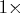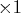Previous Page | Next Page

 Language Reference

reads observations from a data set

READ <range> <VAR operand> <WHERE(expression)>
<INTO name <[ROWNAME=row-name
COLNAME=column-name]> ;

The inputs to the READ function are as follows:
range
specifies a range of observations.

operand
selects a set of variables.

expression
is evaluated for being true or false.

name
is the name of the target matrix.

row-name
is a character matrix or quoted literal giving descriptive row labels.

column-name
is a character matrix or quoted literal giving descriptive column labels.
The clauses and options are explained in the following lists.

Use the READ statement to read variables or records from the current SAS data set into column matrices of the VAR clause or into the single matrix of the INTO clause. When the INTO clause is used, each variable in the VAR clause becomes a column of the target matrix, and all variables in the VAR clause must be of the same type. If you specify no VAR clause, the default variables for the INTO clause are all numeric variables. Read all character variables into a target matrix by using VAR _CHAR_.

You can specify a range of observations with a keyword or by record number using the POINT option. You can use any of the following keywords to specify a range:

ALL
all observations

CURRENT
the current observation

NEXT <number>
the next observation or the next number of observations

AFTER
all observations after the current one

POINT operand
observations specified by number, where operand can be one of the following.

 Operand Example a single record number point 5 a literal giving several point {2 5 10} record numbers the name of a matrix point p containing record numbers an expression in parentheses point (p+1)
If the current data set has an index in use, the POINT option is invalid.

You can specify a set of variables to use with the VAR clause. The operand in the VAR clause can be one of the following:
• a literal containing variable names
• the name of a matrix containing variable names
• an expression in parentheses yielding variable names
• one of keywords described in the following list:
_ALL_
for all variables

_CHAR_
for all character variables

_NUM_
for all numeric variables.
The following examples demonstrate each possible way you can use the VAR clause.

var {time1 time5 time9}; /* a literal giving the variables */
var time;                /* a matrix containing the names  */
var('time1':'time9');    /* an expression                  */
var _all_;               /* a keyword                      */

The WHERE clause conditionally selects observations, within the range specification, according to conditions given in the clause. The general form of the WHERE clause is as follows:

WHERE( variable comparison-op operand)

In the preceding statement,
variable
is a variable in the SAS data set.

comparison-op
is one of the following comparison operators:

<
less than

<=
less than or equal to

=
equal to

>
greater than

>=
greater than or equal to

^=
not equal to

?
contains a given string

^?
does not contain a given string

=:
begins with a given string

=*
sounds like or is spelled like a given string

operand
is a literal value, a matrix name, or an expression in parentheses.
WHERE comparison arguments can be matrices. For the following operators, the WHERE clause succeeds if all the elements in the matrix satisfy the condition:

 ^=   ^?   <   <=   >   >=

For the following operators, the WHERE clause succeeds if any of the elements in the matrix satisfy the condition:

 =   ?   =:   =*

Logical expressions can be specified within the WHERE clause by using the AND (&) and OR (|) operators. The general form is

 clause&clause (for an AND clause) clause|clause (for an OR clause)

where clause can be a comparison, a parenthesized clause, or a logical expression clause that is evaluated by using operator precedence.

Note: The expression on the left-hand side refers to values of the data set variables, and the expression on the right-hand side refers to matrix values.

You can specify ROWNAME= and COLNAME= matrices as part of the INTO clause. The COLNAME= matrix specifies the name of a new character matrix to be created. This COLNAME= matrix is created in addition to the target matrix of the INTO clause and contains variable names from the input data set corresponding to columns of the target matrix. The COLNAME= matrix has dimensionnvar, where nvar is the number of variables contributing to the target matrix.

The ROWNAME= option specifies the name of a character variable in the input data set. The values of this variable are put in a character matrix with the same name as the variable. This matrix has the dimension nobs, where nobs is the number of observations in the range of the READ statement.

The range, VAR, WHERE, and INTO clauses are all optional and can be specified in any order.

Row and column names created via a READ statement are permanently associated with the INTO matrix. You do not need to use a MATTRIB statement to get this association.

For example, to read all observations from the data set variables NAME and AGE, use a READ statement with the VAR clause and the keyword ALL for the range operand. This creates two IML variables with the same names as the data set variables. Here is the statement:

read all var{name age};

To read all variables for the 23rd observation only, use the following statement: